# Please be Seated Dr Enas Abu Taleb Lecturer

• Slides: 25Dr. Enas Abu Taleb Lecturer Basic Sciences Department 2011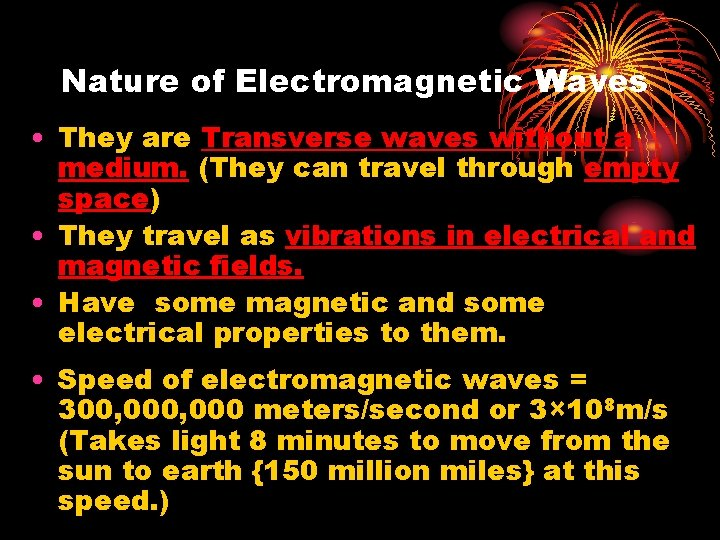Nature of Electromagnetic Waves • They are Transverse waves without a medium. (They can travel through empty space) • They travel as vibrations in electrical and magnetic fields. • Have some magnetic and some electrical properties to them. • Speed of electromagnetic waves = 300, 000 meters/second or 3× 108 m/s (Takes light 8 minutes to move from the sun to earth {150 million miles} at this speed. )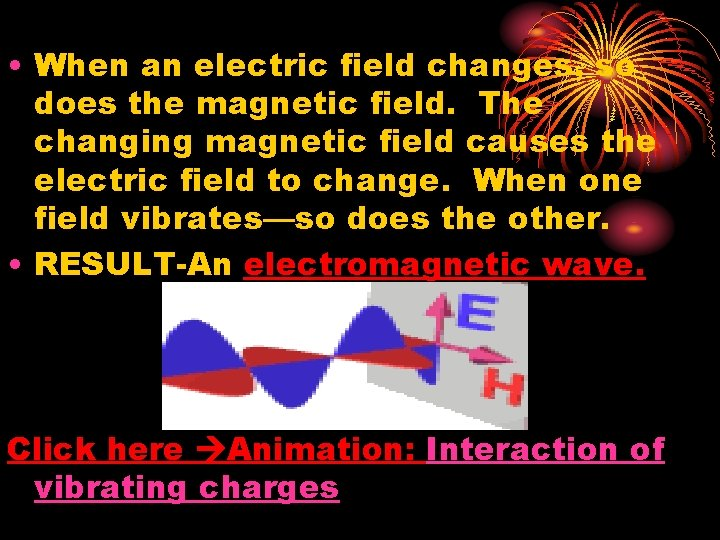• When an electric field changes, so does the magnetic field. The changing magnetic field causes the electric field to change. When one field vibrates—so does the other. • RESULT-An electromagnetic wave. Click here Animation: Interaction of vibrating charges• The electromagnetic spectrum is the distribution of electromagnetic radiation according to energy (or equivalently according to frequency or wavelength). • Electromagnetic radiation covers a broad spectrum of wave lengths and frequencies, ranging from the long wave radio-TV, and short wave diathermy bands.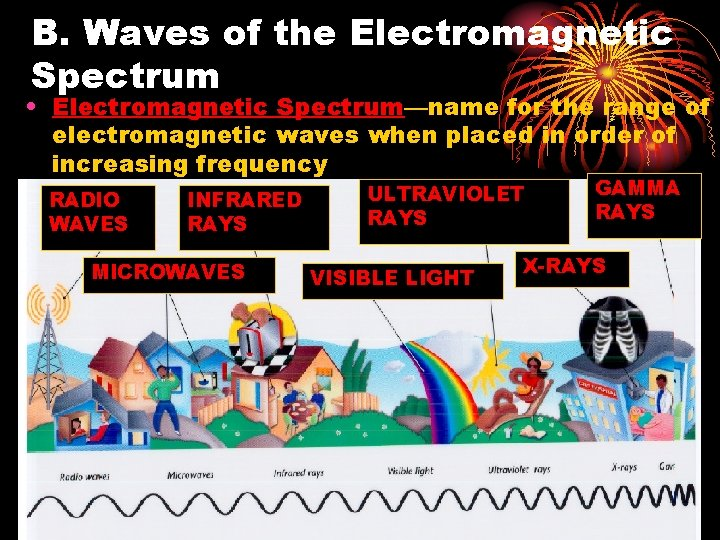B. Waves of the Electromagnetic Spectrum • Electromagnetic Spectrum—name for the range of electromagnetic waves when placed in order of increasing frequency RADIO WAVES INFRARED RAYS MICROWAVES ULTRAVIOLET RAYS VISIBLE LIGHT GAMMA RAYS X-RAYS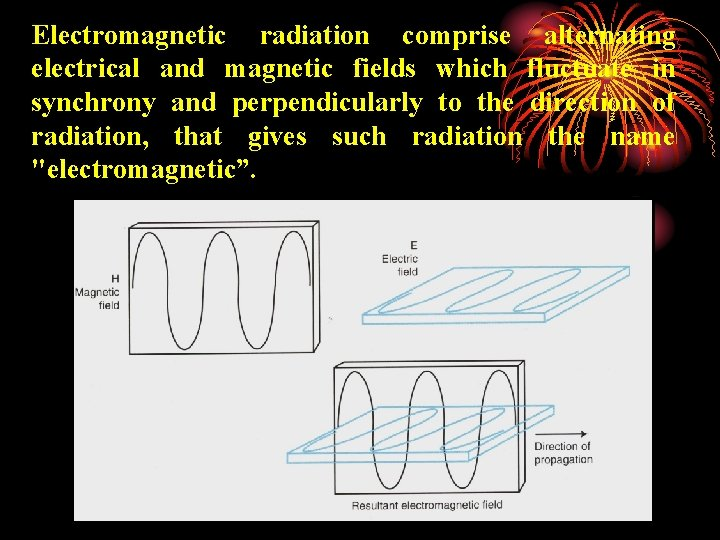Electromagnetic radiation comprise alternating electrical and magnetic fields which fluctuate in synchrony and perpendicularly to the direction of radiation, that gives such radiation the name "electromagnetic”.• Wave length (λ): is the horizontal distance between two adjacent wave peaks (between a point on one electromagnetic wave and exactly the same point on the next wave) • Wave frequency (f): is the number of cycles that occurs in one second. It is measured in Hertz (Hz) • Velocity of light (v) = frequency (f) × wave length (λ) v= λ x f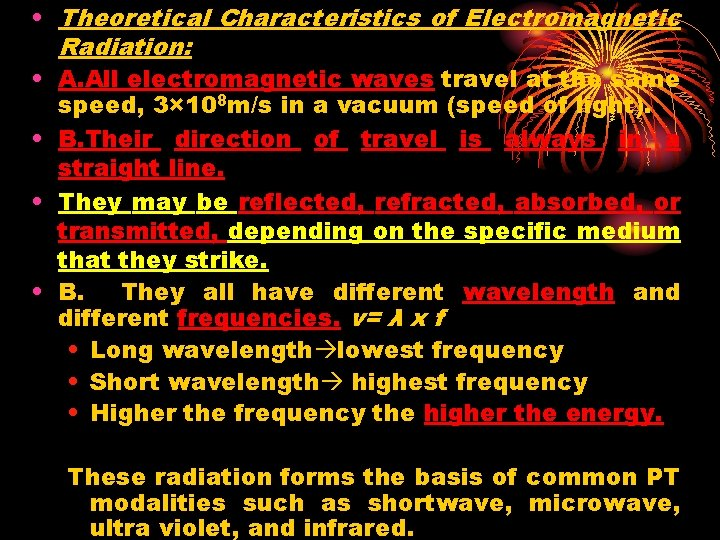• Theoretical Characteristics of Electromagnetic Radiation: • A. All electromagnetic waves travel at the same speed, 3× 108 m/s in a vacuum (speed of light). • B. Their direction of travel is always in a straight line. • They may be reflected, refracted, absorbed, or transmitted, depending on the specific medium that they strike. • B. They all have different wavelength and different frequencies. v= λ x f • Long wavelength lowest frequency • Short wavelength highest frequency • Higher the frequency the higher the energy. These radiation forms the basis of common PT modalities such as shortwave, microwave, ultra violet, and infrared.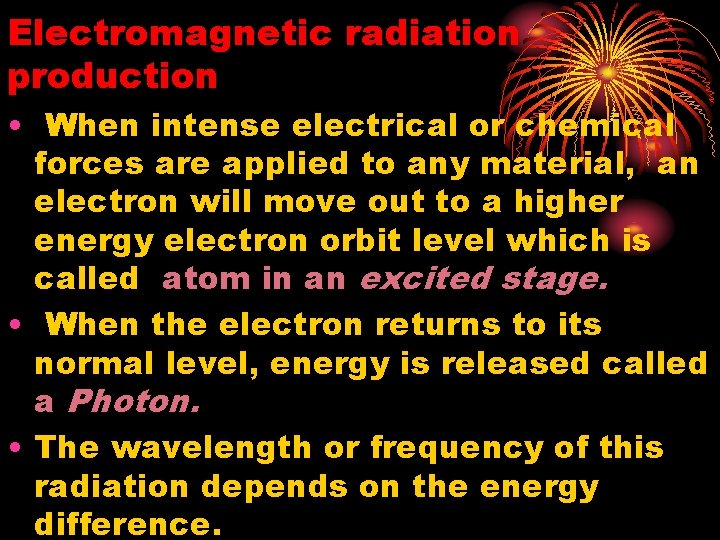Electromagnetic radiation production • When intense electrical or chemical forces are applied to any material, an electron will move out to a higher energy electron orbit level which is called atom in an excited stage. • When the electron returns to its normal level, energy is released called a Photon. • The wavelength or frequency of this radiation depends on the energy difference.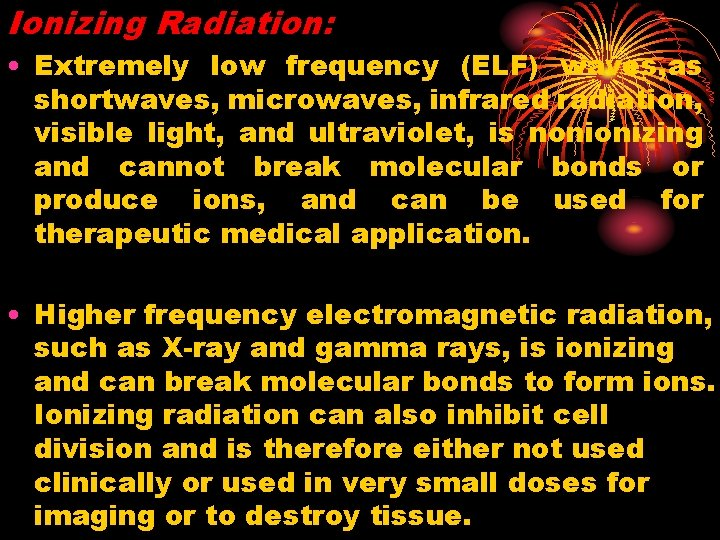Ionizing Radiation: • Extremely low frequency (ELF) waves, as shortwaves, microwaves, infrared radiation, visible light, and ultraviolet, is nonionizing and cannot break molecular bonds or produce ions, and can be used for therapeutic medical application. • Higher frequency electromagnetic radiation, such as X-ray and gamma rays, is ionizing and can break molecular bonds to form ions. Ionizing radiation can also inhibit cell division and is therefore either not used clinically or used in very small doses for imaging or to destroy tissue.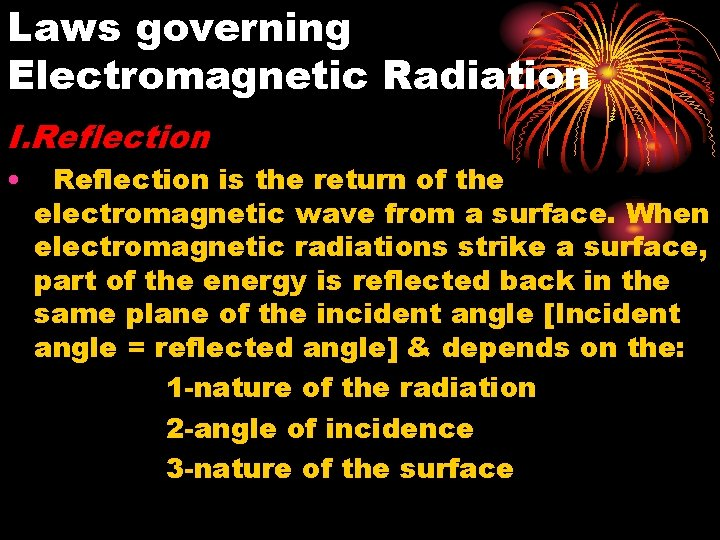Laws governing Electromagnetic Radiation I. Reflection • Reflection is the return of the electromagnetic wave from a surface. When electromagnetic radiations strike a surface, part of the energy is reflected back in the same plane of the incident angle [Incident angle = reflected angle] & depends on the: 1 -nature of the radiation 2 -angle of incidence 3 -nature of the surface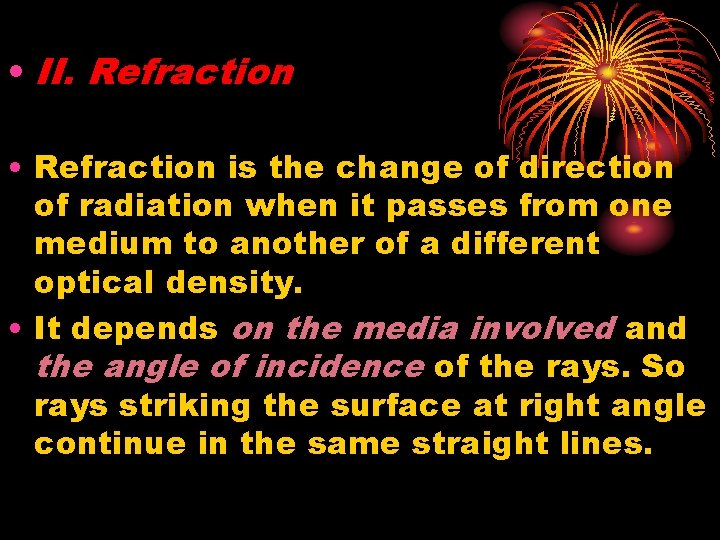• II. Refraction • Refraction is the change of direction of radiation when it passes from one medium to another of a different optical density. • It depends on the media involved and the angle of incidence of the rays. So rays striking the surface at right angle continue in the same straight lines.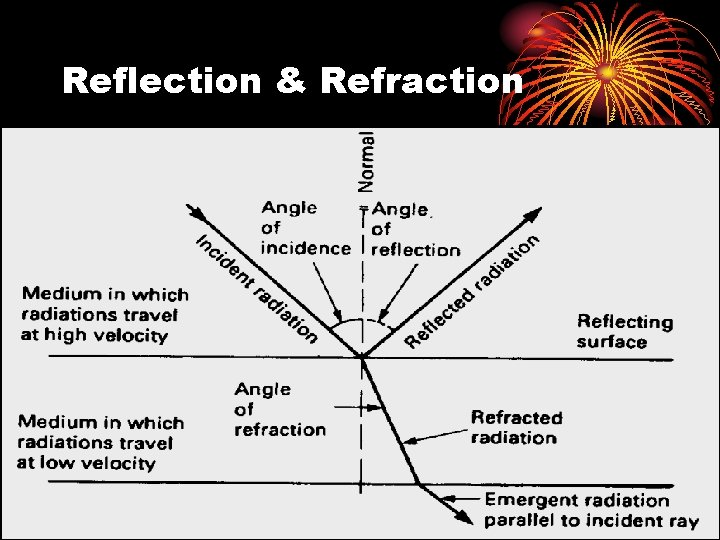Reflection & Refraction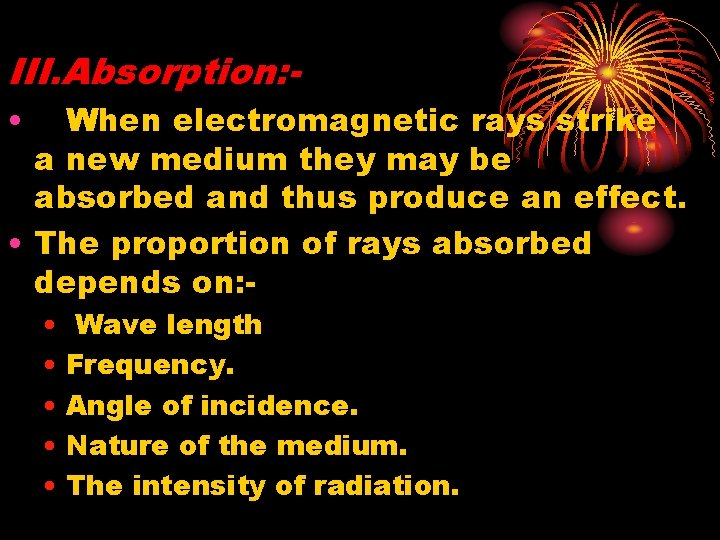III. Absorption: • When electromagnetic rays strike a new medium they may be absorbed and thus produce an effect. • The proportion of rays absorbed depends on: • Wave length • Frequency. • Angle of incidence. • Nature of the medium. • The intensity of radiation.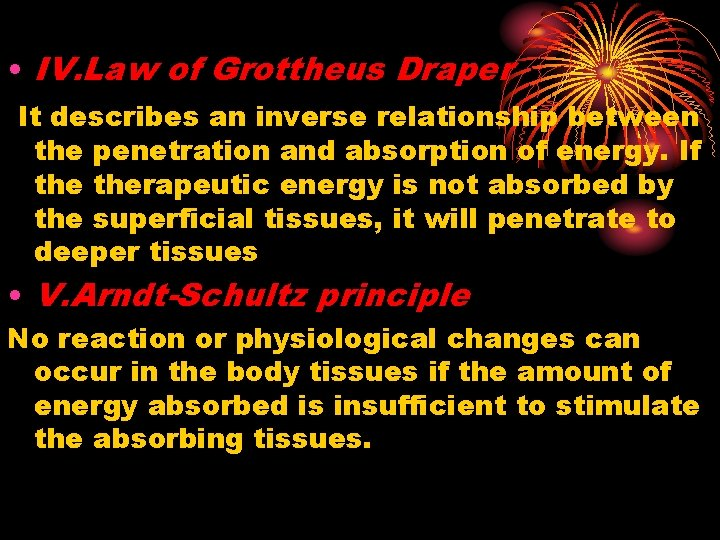• IV. Law of Grottheus Draper It describes an inverse relationship between the penetration and absorption of energy. If therapeutic energy is not absorbed by the superficial tissues, it will penetrate to deeper tissues • V. Arndt-Schultz principle No reaction or physiological changes can occur in the body tissues if the amount of energy absorbed is insufficient to stimulate the absorbing tissues.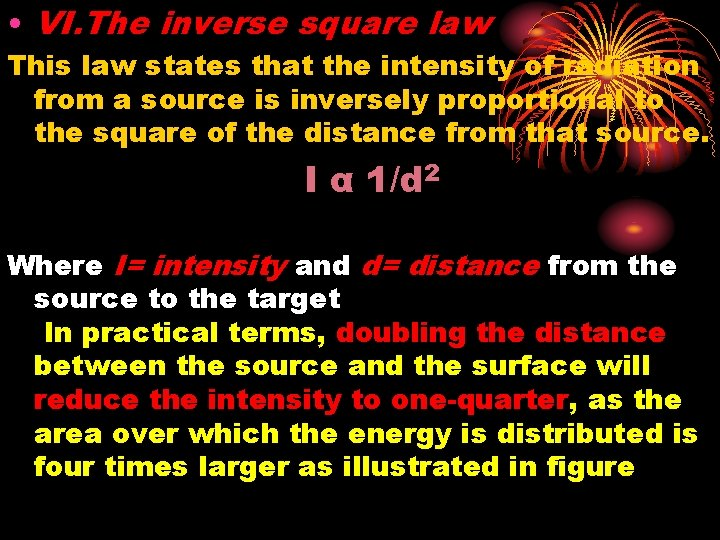• VI. The inverse square law This law states that the intensity of radiation from a source is inversely proportional to the square of the distance from that source. I α 1/d 2 Where I= intensity and d= distance from the source to the target In practical terms, doubling the distance between the source and the surface will reduce the intensity to one-quarter, as the area over which the energy is distributed is four times larger as illustrated in figureThe inverse square law• VII. The Lambert's cosine law This law states that the intensity of radiation varies with the cosine of the angle of incidence. • Effective energy= energy X cosine of the • angle of incidence • Electromagnetic energy is most efficiently transmitted to the tissue when it strikes the body at right angle.• VIII. Scattering Radiation passing through non-homogenous matter maybe partly scattered (the direction of some radiation is altered), reducing the depth of penetration. • Depends on the wave length of radiation. Longer wave lengths are scattered less than shorter ones. The reason is that waves are reflected if their wavelengths are less than the size of the reflecting object, so much energy will be reflected and so be scattered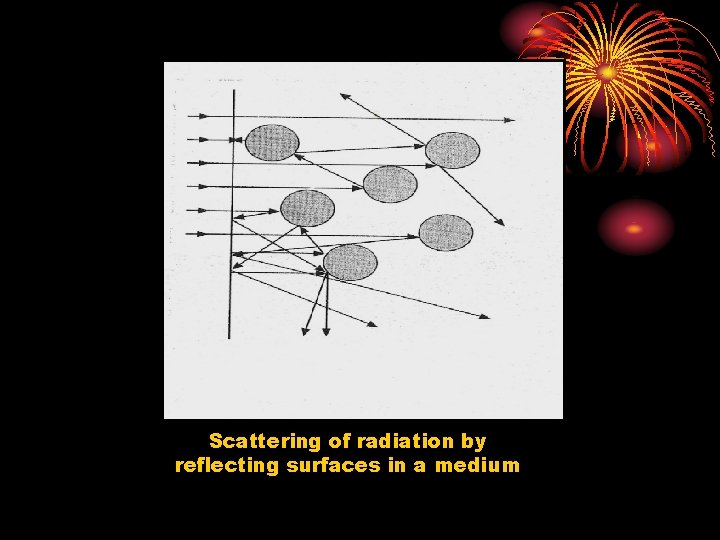Scattering of radiation by reflecting surfaces in a mediumThe End See you Next “Physiological and Physical Effects of Heat”Thank You